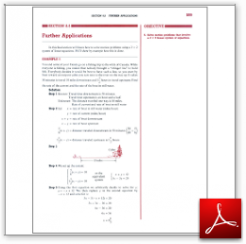Algebra Math Book - Introductory Algebra Thank you for your continual support.

 The power of mathematics rests in the logic of thinking.ID: Sec8-5
Description: Motion and Travel
Price: 1.99

# Algebra Math book - Introductory Algebra - Chapter 8 - Section 5 - Further Applications

## Section 8.5 - Objectives

5.  Solve motion problems that involve a 2 x 2 linear system of equations.

Navigate to
Previous Section or Next Chapter
or Chapter 8 Details or Other Chapters

This section of my algebra math book, Introductory Algebra, also includes in the download:

• Cover Sheet